# 1 Short pulse neutron source Pulse length 1

• Slides: 10
Download presentation1 - Short pulse neutron source Pulse length: ~ 1 s Repetition rate: 50 – 60 Hz Average beam power: ~ 1. 5 MW Beam energy: 1 – 8 Ge. V Particle type: protons or H- Spallation Neutron Source (ORNL)Overview 3 Me. V H- source LEBT RFQ MEBT 90 Me. V DTL 200 Me. V CCL 1 Ge. V SCL HEBT Storage Ring 352. 2 MHz 15 m 704. 4 MHz 400 m Target Wf = 1 Ge. V, If = 1. 5 m. A (average), then P = 1. 5 MW. Average ion source current estimated to be Is = 2 -2. 5 m. A (in order to account for transverse and longitudinal losses along the LINAC, as well as chopped portions of the beam). Repetition rate = 50 Hz, Duty Factor = 6%, then Is = 33 -42 m. A (peak).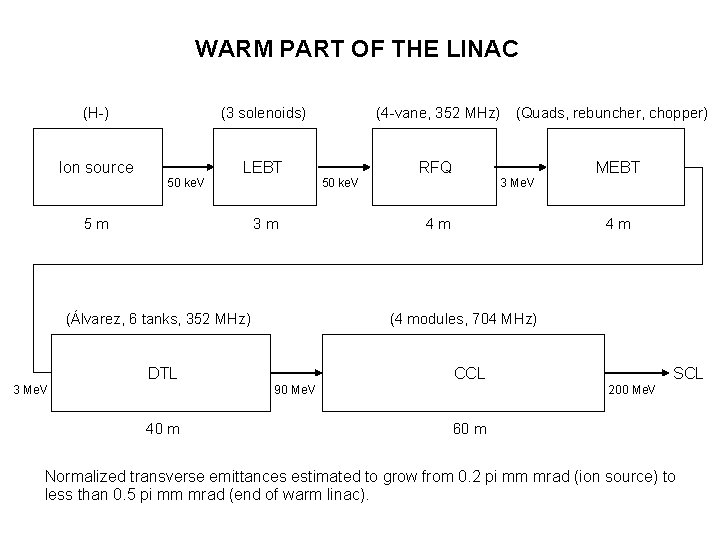WARM PART OF THE LINAC (H-) Ion source (3 solenoids) 50 ke. V LEBT 5 m 3 m (Álvarez, 6 tanks, 352 MHz) (4 -vane, 352 MHz) 50 ke. V RFQ 3 Me. V 4 m 4 m CCL 90 Me. V 40 m MEBT (4 modules, 704 MHz) DTL 3 Me. V (Quads, rebuncher, chopper) SCL 200 Me. V 60 m Normalized transverse emittances estimated to grow from 0. 2 pi mm mrad (ion source) to less than 0. 5 pi mm mrad (end of warm linac).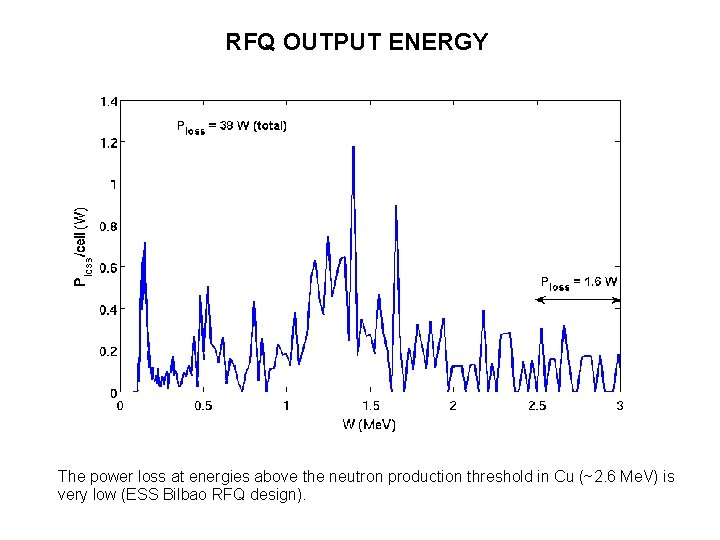RFQ OUTPUT ENERGY The power loss at energies above the neutron production threshold in Cu (~2. 6 Me. V) is very low (ESS Bilbao RFQ design).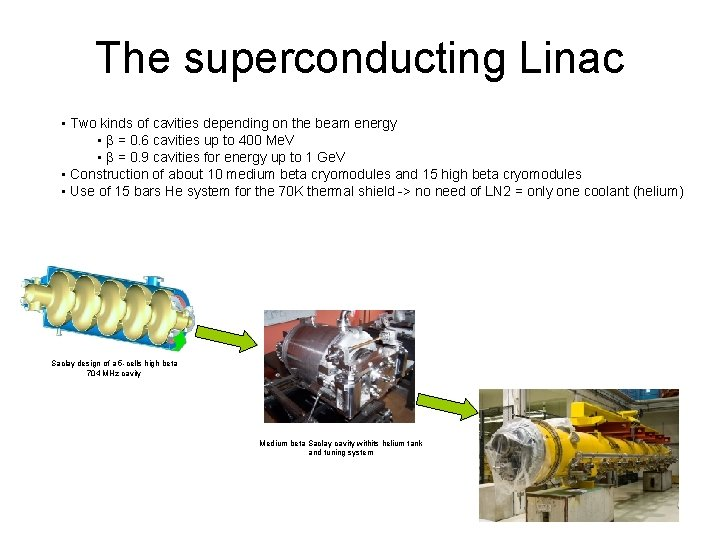The superconducting Linac • Two kinds of cavities depending on the beam energy • b = 0. 6 cavities up to 400 Me. V • b = 0. 9 cavities for energy up to 1 Ge. V • Construction of about 10 medium beta cryomodules and 15 high beta cryomodules • Use of 15 bars He system for the 70 K thermal shield -> no need of LN 2 = only one coolant (helium) Saclay design of a 5 -cells high beta 704 MHz cavity Medium beta Saclay cavity withits helium tank and tuning systemStorage ring Beam rigidity: Magnetic field B = 0. 617 T → 8 Ge. V 1 Ge. V Radius = 9. 168 m → Circumference Circ. = 57. 6 m Only dipoles! We need more space for other elements. Arc section: 90 m, Straight section: 90 m, Total circumference: 180 m Arc section Cells: 12 → Cell length: 7. 5 m, Dipoles/cell: 2 → Total dipoles: 24 angle = 360/24 = 15° Arc section - 3 FODO cells → dipole length = 2. 4 m sector dipole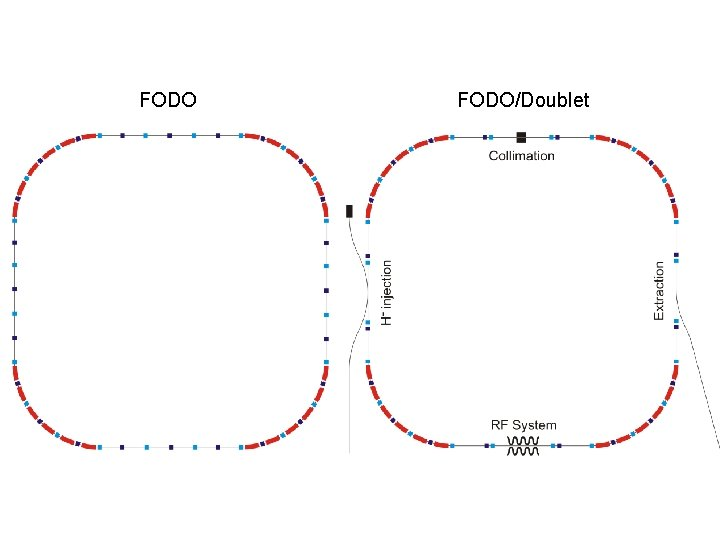FODO/Doublet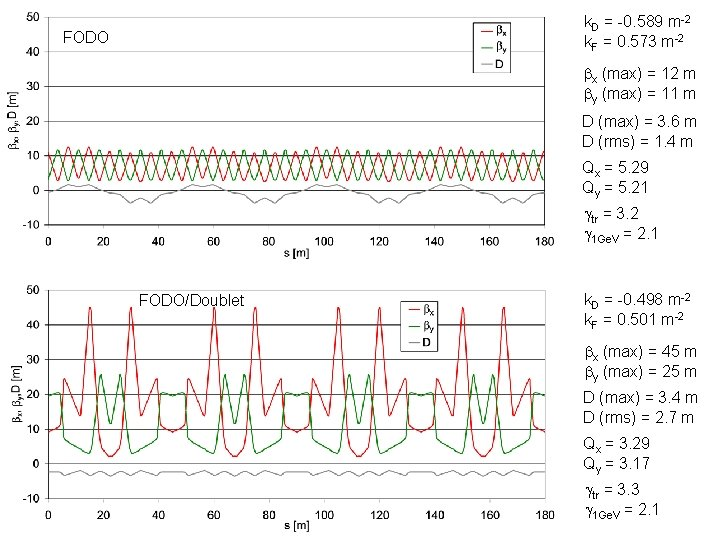k. D = -0. 589 m-2 k. F = 0. 573 m-2 FODO bx (max) = 12 m by (max) = 11 m D (max) = 3. 6 m D (rms) = 1. 4 m Qx = 5. 29 Qy = 5. 21 gtr = 3. 2 g 1 Ge. V = 2. 1 FODO/Doublet k. D = -0. 498 m-2 k. F = 0. 501 m-2 bx (max) = 45 m by (max) = 25 m D (max) = 3. 4 m D (rms) = 2. 7 m Qx = 3. 29 Qy = 3. 17 gtr = 3. 3 g 1 Ge. V = 2. 1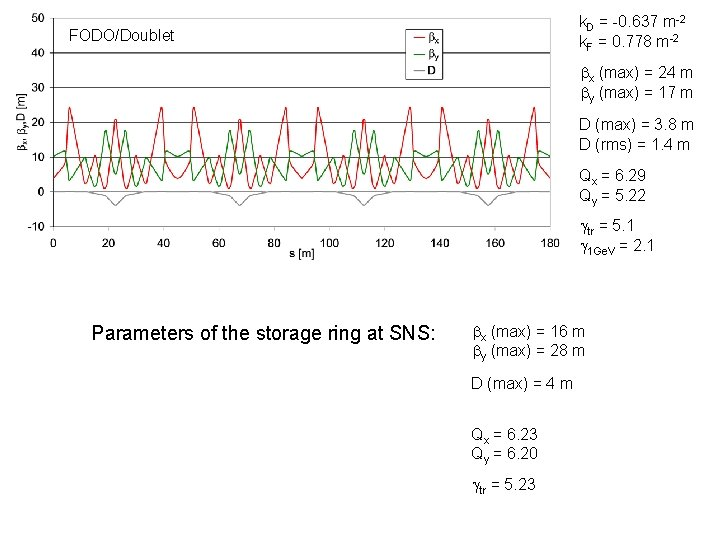k. D = -0. 637 m-2 k. F = 0. 778 m-2 FODO/Doublet bx (max) = 24 m by (max) = 17 m D (max) = 3. 8 m D (rms) = 1. 4 m Qx = 6. 29 Qy = 5. 22 gtr = 5. 1 g 1 Ge. V = 2. 1 Parameters of the storage ring at SNS: bx (max) = 16 m by (max) = 28 m D (max) = 4 m Qx = 6. 23 Qy = 6. 20 gtr = 5. 23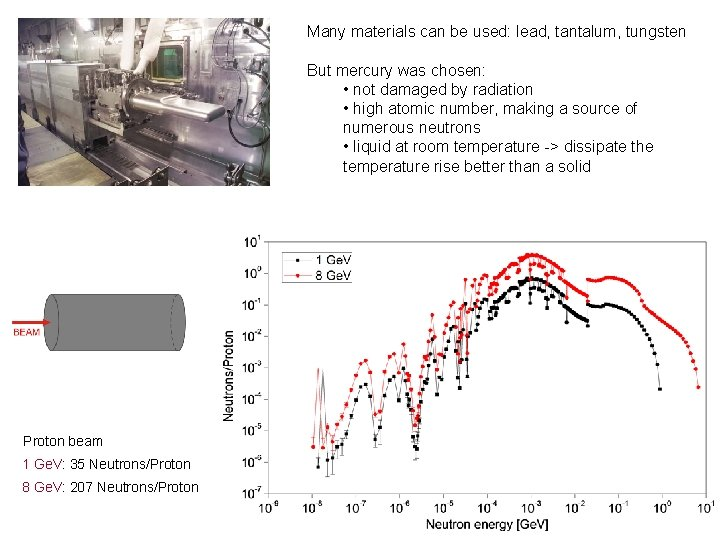Many materials can be used: lead, tantalum, tungsten But mercury was chosen: • not damaged by radiation • high atomic number, making a source of numerous neutrons • liquid at room temperature -> dissipate the temperature rise better than a solid Proton beam 1 Ge. V: 35 Neutrons/Proton 8 Ge. V: 207 Neutrons/Proton